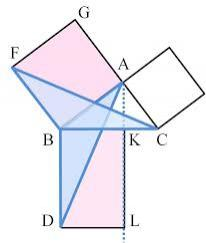# Euclid 5

Calculate the length of remain sides of a right triangle ABC if a = 7 cm and height vc = 5 cm.

Result

b =  7.14 cm
c =  10 cm

#### Solution:

$c = c_1 +c_2 \ \\ c_1^2 = a^2 -v^2 \ \\ c_1 = \sqrt{ 7^2 - 5^2 } = 4.9 \ cm \ \\ v^2 = c_1 c_2 \ \\ c_2 = v^2/c_1 = 5^2 / 4.9 = 5.1 \ cm \ \\ \ \\ c = c_1 + c_2 = 10 \ \text{cm} \ \\ \ \\ c^2 = a^2+b^2 \ \\ b = \sqrt{ c^2 - a^2 } = 7.14 \ cm$

Try calculation via our triangle calculator.Our examples were largely sent or created by pupils and students themselves. Therefore, we would be pleased if you could send us any errors you found, spelling mistakes, or rephasing the example. Thank you!

Leave us a comment of this math problem and its solution (i.e. if it is still somewhat unclear...):Be the first to comment!Tips to related online calculators
Pythagorean theorem is the base for the right triangle calculator.

#### You need to know the following knowledge to solve this word math problem:

We encourage you to watch this tutorial video on this math problem:

## Next similar math problems:

1. Euclid theoremsCalculate the sides of a right triangle if leg a = 6 cm and a section of the hypotenuse, which is located adjacent the second leg b is 5cm.
2. Without Euclid lawsRight triangle ABC with right angle at the C has a=5 and hypotenuse c=19. Calculate the height h of this triangle without the use of Euclidean laws.
3. Euclidean distanceCalculate the Euclidean distance between shops A, B and C, where: A 45 0.05 B 60 0.05 C 52 0.09 Wherein the first figure is the weight in grams of bread and second figure is price in USD.
4. Squares above sidesTwo squares are constructed on two sides of the ABC triangle. The square area above the BC side is 25 cm2. The height vc to the side AB is 3 cm long. The heel P of height vc divides the AB side in a 2: 1 ratio. The AC side is longer than the BC side. Calc
5. Medians in right triangleIt is given a right triangle, angle C is 90 degrees. I know it medians t1 = 8 cm and median t2 = 12 cm. .. How to calculate the length of the sides?
6. Euklid4Legs of a right triangle have dimensions 244 m and 246 m. Calculate the length of the hypotenuse and the height of this right triangle.
7. CirclesIn the circle with a radius 7.5 cm are constructed two parallel chord whose lengths are 9 cm and 12 cm. Calculate the distance of these chords (if there are two possible solutions write both).
8. Rhombus and inscribed circleIt is given a rhombus with side a = 6 cm and the radius of the inscribed circle r = 2 cm. Calculate the length of its two diagonals.
9. RT triangle and heightCalculate the remaining sides of the right triangle if we know side b = 4 cm long and height to side c h = 2.4 cm.
10. Isosceles IVIn an isosceles triangle ABC is |AC| = |BC| = 13 and |AB| = 10. Calculate the radius of the inscribed (r) and described (R) circle.
11. Triangle ABCIn a triangle ABC with the side BC of length 2 cm The middle point of AB. Points L and M split AC side into three equal lines. KLM is isosceles triangle with a right angle at the point K. Determine the lengths of the sides AB, AC triangle ABC.
12. Free space in the gardenThe grandfather's free space in the garden was in the shape of a rectangular triangle with 5 meters and 12 meters in length. He decided to divide it into two parts and the height of the hypotenuse. For the smaller part creates a rock garden, for the large
13. Right isosceles triangleRight isosceles triangle has an altitude x drawn from the right angle to the hypotenuse dividing it into 2 equal segments. The length of one segment is 5 cm. What is the area of the triangle?
14. Right 24Right isosceles triangle has an altitude x drawn from the right angle to the hypotenuse dividing it into 2 unequal segments. The length of one segment is 5 cm. What is the area of the triangle? Thank you.
15. RT sidesFind the sides of a rectangular triangle if legs a + b = 17cm and the radius of the written circle ρ = 2cm.
16. Leg and heightSolve right triangle with height v = 9.6 m and shorter cathetus b = 17.3 m.
17. Isosceles triangle 9Given an isosceles triangle ABC where AB= AC. The perimeter is 64cm and altitude is 24cm. Find the area of the isosceles triangle